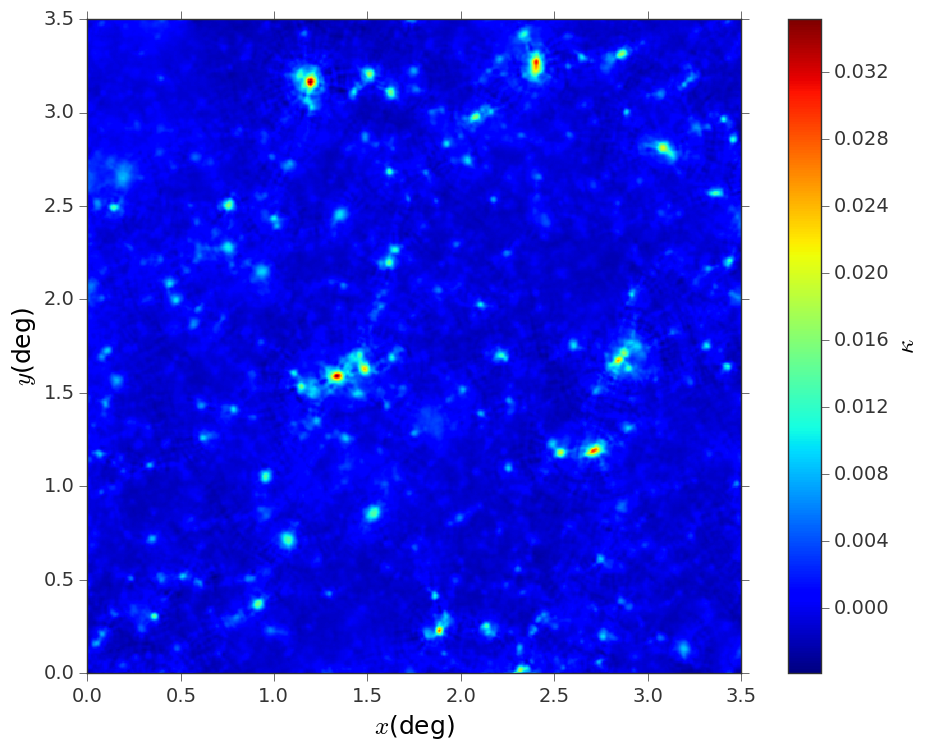# Construct convergence maps out of shear catalogs¶

Constructing a convergence map of a particular subfield from a shear catalog is an operation that occurs frequently in weak lensing analysis; if the field of view is unmasked, the reconstruction operation is equivalent to the calculation of the $$E$$ mode of the shear field $$\gamma$$. Here is how to to use lenstools to perform this operation: the operations are handled with the ShearCatalog class

from lenstools.catalog.shear import ShearCatalog
import astropy.table as tbl
import astropy.units as u


Suppose that the shear table is contained in a file called ‘WLshear.fits’ (which should contain a table with columns ‘shear1’,’shear2’ readable with astropy.table.Table.read()), in which each row represents a different galaxy; suppose also that the sky positioning information $$(x,y)$$ is contained in a file ‘positions.fits’; we must combine the information in these two tables to build a $$(x,y,\gamma)$$ table

shear_catalog = ShearCatalog.read('WLshear.fits')
full_catalog = tbl.hstack((positions_catalog,shear_catalog))


You can tweak the names of the columns that contain the position information (which by default are ‘x’,’y’) and the measure units of the positions (which by default are degrees) using the setSpatialInfo() method. At this point you can construct a shear grid out of the catalog, specifying a grid size and a smoothing scale.

shear_map = full_catalog.toMap(map_size=3.5*u.deg,npixel=512,smooth=0.5*u.arcmin)
convergence_map = shear_map.convergence()


Note that ‘shear_map’ now is a ShearMap instance and ‘convergence_map’ is a ConvergenceMap instance, and as such you can visualize it with the visualize() method

convergence_map.visualize(colorbar=True,cbar_label=r"$\kappa$")